# Test Prep Plan - Take a practice test

Take this practice test to check your existing knowledge of the course material. We'll review your answers and create a Test Prep Plan for you based on your results.
How Test Prep Plans work
1
2Based on your results, we'll create a customized Test Prep Plan just for you!
3Study smarter
Study more effectively: skip concepts you already know and focus on what you still need to learn.

# NMTA Math: Limits Chapter Exam

Exam Instructions:

Choose your answers to the questions and click 'Next' to see the next set of questions. You can skip questions if you would like and come back to them later with the yellow "Go To First Skipped Question" button. When you have completed the practice exam, a green submit button will appear. Click it to see your results. Good luck!

### Page 1

#### Question 1 1. Use the graph to solve for z.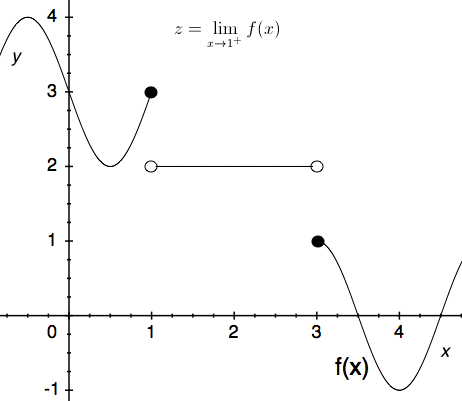#### Question 2 2. The ceiling function is graphically translated as a stepwise function. What is the limit of f(x) = ceil(x) as x approaches 4.5?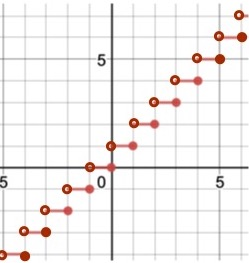#### Question 3 3. Use the given formula to solve for z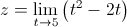#### Question 5 5. Which of the following is true?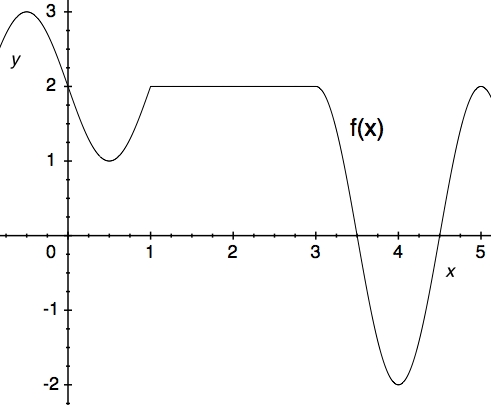### Page 2

#### Question 6 6. Using the given data, solve for the continuous function y.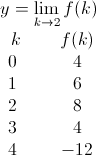#### Question 7 7. The floor function is graphically represented as a stepwise function. What is the limit of f(x) = floor(x) as x approaches 4?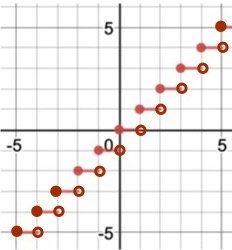#### Question 8 8. Use the graph to solve for z.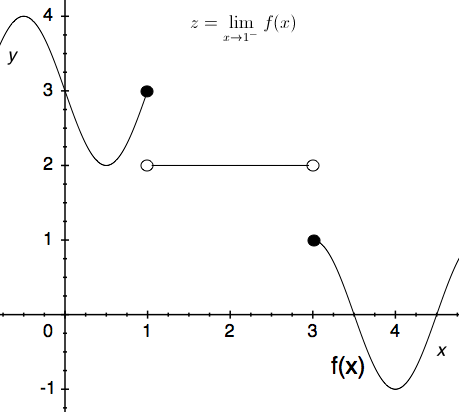#### Question 10 10. Use the graph to solve for z.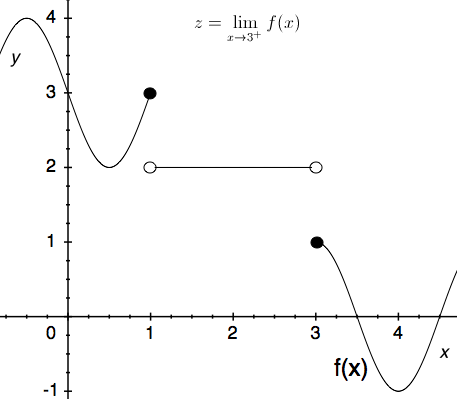### Page 3

#### Question 11 11. Use the given graph and formula to solve for z.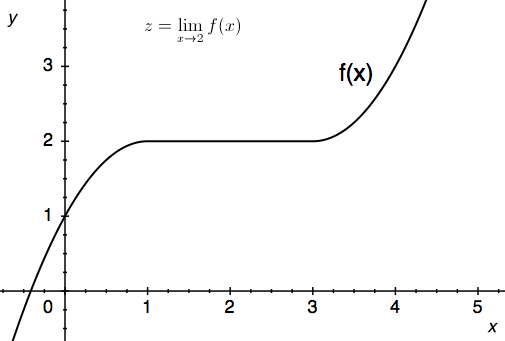#### Question 12 12. Use the given graph and formula to solve for z.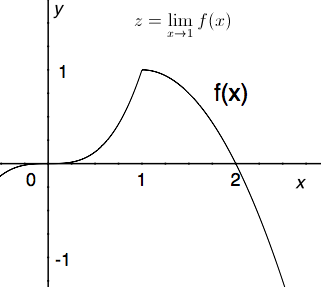### Page 4

#### Question 16 16. Use the graph to solve for z.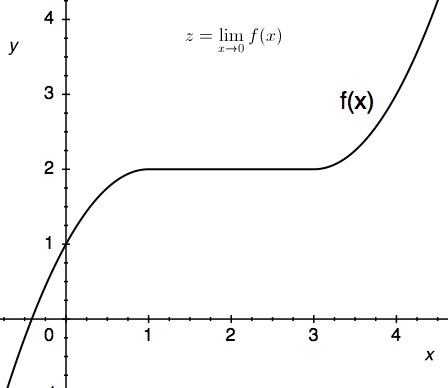#### Question 18 18. Using the graph, find the value of z, then add 1.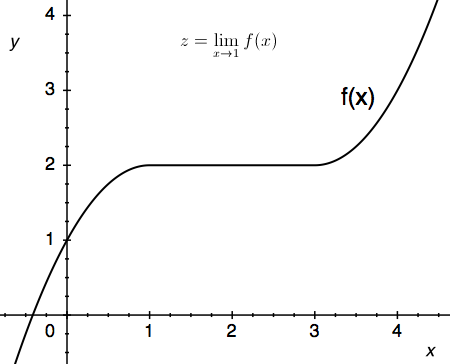#### Question 19 19. Solve for the limit: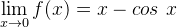#### Question 20 20. Using the given data, predict the likely value of the continuous function z.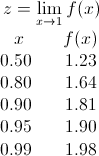#### NMTA Math: Limits Chapter Exam Instructions

Choose your answers to the questions and click 'Next' to see the next set of questions. You can skip questions if you would like and come back to them later with the yellow "Go To First Skipped Question" button. When you have completed the practice exam, a green submit button will appear. Click it to see your results. Good luck!

Support